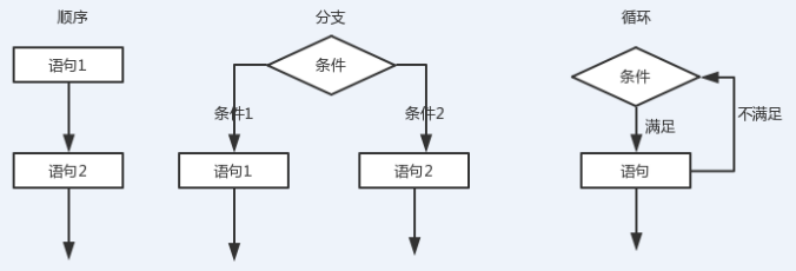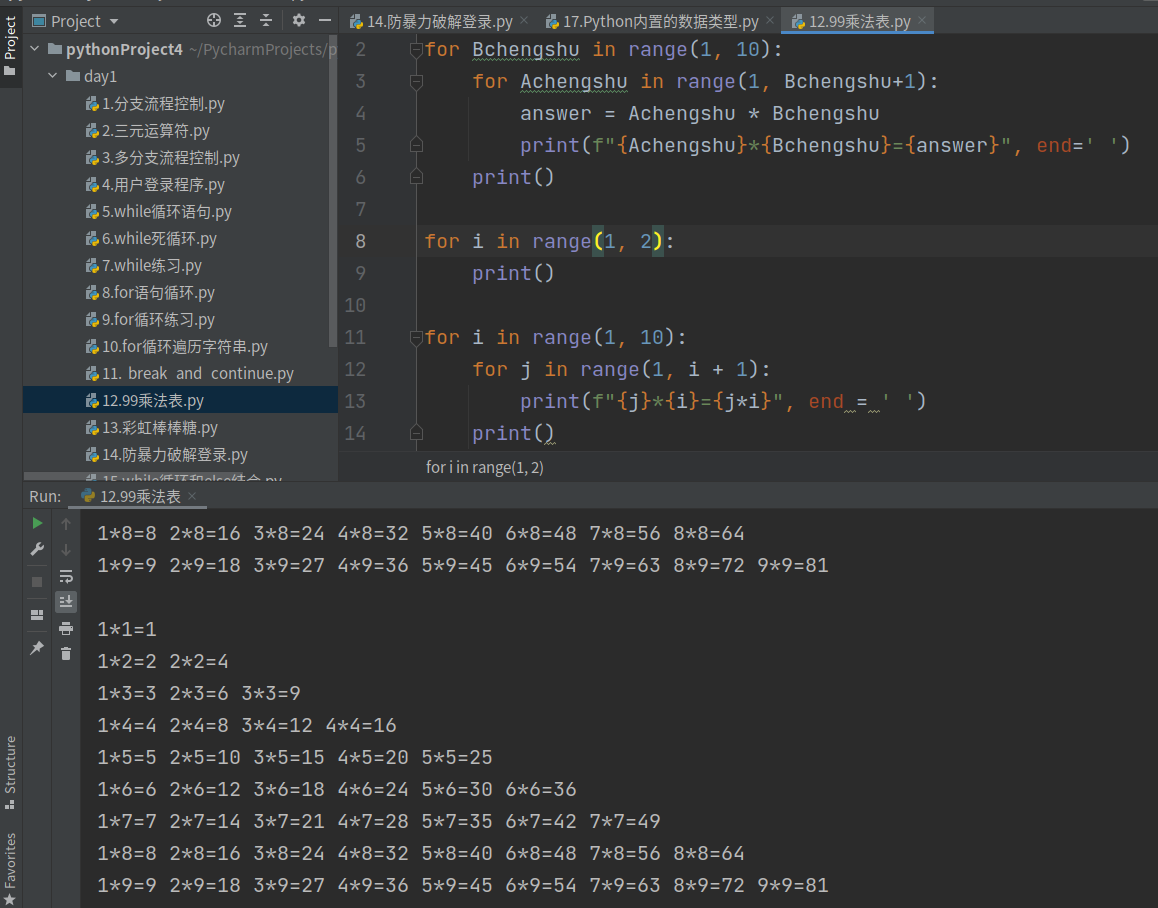## Python 基础知识整理详解（常见数据类型，标准输入输出，三大流程控制语句，跳出循环，乘法表等实例）

2021/2/6 22:20:45 文章标签: 测试文章如有侵权请发送至邮箱809451989@qq.com投诉后文章立即删除

1.Python 常见数据类型&#xff1a; int &#xff1a; 整数&#xff0c;1&#xff0c;2&#xff0c;90 float &#xff1a; 浮点数&#xff0c;3.1313&#xff0c; 2e-2&#xff08;0.02&#xff09;,2e4(20000) bool&#xff1a;逻辑值真假&#xff08;True &#xff0c;Flas…

1.Python 常见数据类型：

int ： 整数，1，2，90
float ： 浮点数，3.1313， 2e-2（0.02）,2e+4(20000)
bool：逻辑值真假（True ，Flase）

+， -， *， /， **（次方）， %（取余）， //取整

=，+=，-=，*=，/=，，**=，%=，//=

``````>>> num = 1
>>> num += 1 #num = num+1
>>> num
2
``````

（返回的值为bool值） > , >=，< ,<=, !=, ==(判断值是否相等)

&(and) ，| (or) ，! (not)

2.标准输入输出：

age = int（input（“年龄：”））

print（“姓名：%s，年龄：%d，分数：%.2f” %(name, age, score)）;
print (f"姓名：{name}, 年龄：{age}, 分数：{score}")

3.常见函数与导入常用模块：

``````>>> abs(-23)
23
>>> divmod(10,3)
(3, 1)
>>> round(3.16245,4)
3.1625
>>> pow(3,4)
81

``````

``````>>> import math
>>> math.pi
3.141592653589793
>>> math.sqrt(16)
4.0
>>> import random
>>> random.randint(1,100)
32
>>> random.randint(1,100)
74
``````

``````name = input("姓名：")
yuwen = int(input("语文成绩："))
shuxue = int(input("数学成绩："))
yingyu = int(input("英语成绩："))
zongfen = float(yuwen+shuxue+yingyu)
pingjun = zongfen/3
print("%s同学的总分为%.2f，平均成绩为%.2f" %(name,zongfen,pingjun))

``````

4.三大流程控制语句：1. 单分支：
if 条件 ：
满足条件执行的内容

2. 双分支：
if 条件 ：
满足条件执行的内容
else ：
不满足条件执行的内容

（也可以用三元运算符实现： 满足条件的内容 if 条件 else 不满足条件的内容）

1. 多分支：
if 条件1 ：
满足条件1执行的内容
elif 条件2 ：
满足条件2执行的内容
else：
不满足条件执行的内容

``````age = int(input("nian ling: "))
if age > 18:
print("cheng nian")
else:
print("wei cheng nian")
``````

``````score = int(input("cehngji:"))
if 90<=score<=100:
print("level A")
elif 80<=score<90:
print("level B")
elif 0<=score<80:
print("level C")
else:
print("input error")
``````

`

`

while循环：

``````while 循环语句：  while  条件 ：
count = 0
while count <= 100 :
print(count)
count += 1

while 死循环 ： while True ：
try_count = 0
while True:
print(f"用户第{try_count}次登录")
try_count += 1
user = input("输入用户名:")
passwd = input("输入密码:")
if user == "admin" and passwd == "westos":
print(f"用户{user}登录成功")
exit()
else:
print(f"用户{user}登录失败")

``````

for 循环：

``````1： for I  in  range（start，end，step）

range(start,end) 从 start 开始，到end-1 结束，start没有时，默认为0, step 为部长 ；

for num in range(0,11):
print(num)

0到100之间的偶数：
for num in range(0,101,2):
print(num)

2： for item  in  string：

string = 'westoslinux'
for item in string:
print(“---”，item)

``````

``````
break： 遇到break循环结束
continue： 遇到continue 跳出本次循环

count = 0
while count <= 10 :
print(count)
count += 1
if count == 6 :
break

for item in range(0,11):
if item != 6 :
print("---", item)
continue
pirnt("@@",item)

exit（）：结束程序 ；
````````````for Bchengshu in range(1, 10):
for Achengshu in range(1, Bchengshu+1):
print()
``````

`

`
5：Python内置的数据类型：

``````"""

"""
# 1. 字符串str
s1 = 'hello'
s2 = "hello"
s3 = """
"""
print(type(s1), type(s2), type(s3))

# 2. 列表List
li1 = [1, 2, 3, 4]
print(li1, type(li1))
li2 = [1, 2.4, True, 2e+5, [1, 2, 3]]
print(li2, type(li2))

# 3. 元组tuple
# 易错点: 如果元组只有一个元素，一定要加逗号。
t1 = (1, 2.4, True, 2e+5, [1, 2, 3])
print(t1, type(t1))
t2 = (1,)
print(t2, type(t2))

# 4. 集合set(无序，不重复)
set1 = {1, 2, 1, 2, 3, 1, 20}
print(set1)   # 不重复{1, 2, 20}
set2 = {1, 2, 3}
set3 = {2, 3, 4}
print("交集:", set2 & set3)
print("并集:", set2 | set3)

# 5. 字典dict： {“name”:"westos", "age":10}
# key和value, 键值对， 通过key可以快速找到value值。
user = {"name":'westos', 'age':10}
print(user, type(user))
print(user['name'])
print(user['age'])
``````

暂无相关的数据...